# Class 8 Maths NCERT Solutions for Chapter – 8 Comparing Quantities Ex – 8.3

## Comparing Quantities

Question 1.
Calculate the amount and compound interest on
(a)
₹ 10,800 for 3 years at 12$\frac { 1 }{ 2 }$ % per annum compounded annually.
(b) ₹ 18,000 for 2$\frac { 1 }{ 2 }$ years at 10% per annum compounded annually.
(c) ₹ 62,500 for 1$\frac { 1 }{ 2 }$ years at 8% per annum compounded half yearly.
(d) ₹ 8,000 for 1 year at 9% per annum compounded half yearly. (You could use the year by year calculation using SI formula to verify)
(e) ₹ 10,000 for 1 year at 8% per annum compounded half yearly.

Solution: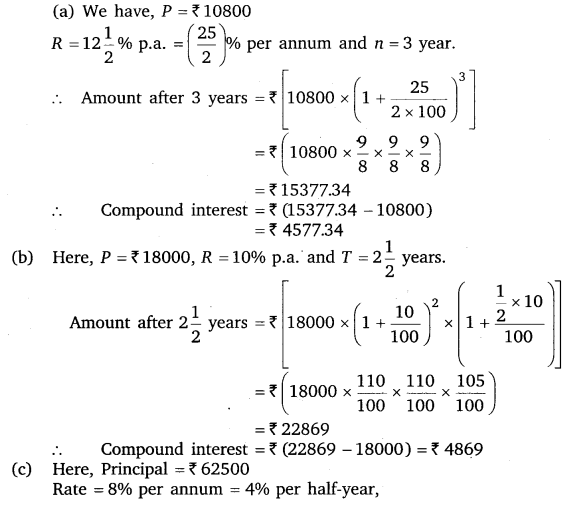Question 2.
Kamala borrowed ₹ 26,400 from a Bank to buy a scooter at a rate of 15% p.a. compounded yearly. What amount will she pay at the end of 2 years and 4 months to clear the loan?
[Hint : Find A for 2 years with interest is compounded yearly and then find SI on the 2nd year amount for$\frac { 4 }{ 12 }$ years)

Solution:
Here, P = ₹ 26400, R =15% per annum
and n = 2 years 4 months =2$\frac { 1 }{ 3 }$ years.Hence, Kamala will pay ₹ 36659.70 to the bank.

Question 3.
Fabina borrows ? 12,500 at 12% per annum for 3 years at simple interest and Radha borrows the same amount for the same time period at 10% per annum, compounded annually. Who pays more interest and by how much? .

Solution:
In case of Fabina :
P = ₹ 12500, R =12% per annum and T =3 years. Then,Hence, Fabina pays 362.50 more as interest ₹ (4500 – 4137.50), i.e., ₹ 362.50 more as interest.

Question 4.
I borrowed ₹ 12,000 from Jamshed at 6% per annum simple interest for 2 years. Had I borrowed this sum at 6% per annum compound interest, what extra amount would I have to pay?

Solution:
Here, P = ₹ 12000, R = 6% per annum and T = 2 years.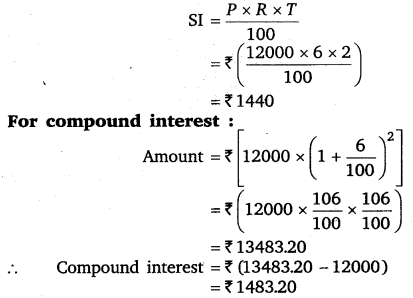So, I have to pay ₹ (1483.20 -1440), i.e., ₹ 43.20 in excess.

Question 5.
Vasudevan invested ₹ 60,000 at an interest rate of 12% per annum compounded half-yearly. What amount would he get
(i)
after 6 months?
(ii) after 1 year.

Solution:
Here, Principal =₹ 60000, Rate = 12% per annum = 6%per half-year.
(i) Time = 6 months = 1 half-year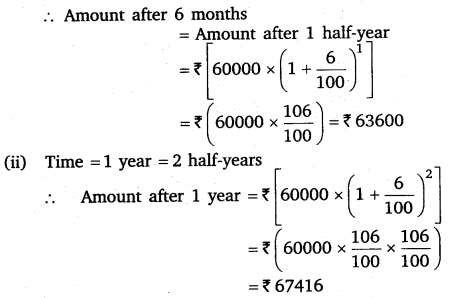Question 6.
Arif took a loan of ? 80,000 from a bank. If the rate of interest is 10% per annum, find the difference in amounts he would be paying after 1$\frac { 1 }{ 2 }$ years if the interest is 2
(i)
compounded annually
(ii) compounded half-yearly.

Solution:
Here, P = ₹ 80000
Rate = 10% per annum = 5% per half-year,
Time = 1$\frac { 1 }{ 2 }$ years = 3 half-years.Question 7.
Maria invested ? 8,000 in a business. She would he paid interest at 5% per annum compounded annually. Find
(i)
The amount credited against her name at the end of the second year.
(ii) The interest for the 3rd year.

Solution: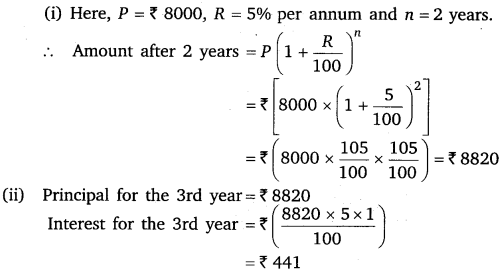Question 8.
Find the amount and the compound interest on ? 10,000 for 1$\frac { 1 }{ 2 }$ years at 10% per annum, compounded half-yearly. Would this interest be more than the interest he would get if it was compounded annually?

Solution:
Here, Principal = ? 10000
Time = 1$\frac { 1 }{ 2 }$ years = 3 half years,This interest is more than the interest that he would get if it was compounded annually.

Question 9.
Find the amount which Ram will get on ₹ 4096, if he gave it for 18 months at 12$\frac { 1 }{ 2 }$ % per annum, interest being compounded half yearly.

Solution:
Here, Principal = ₹ 4096,
Time = 18 months = 3 half years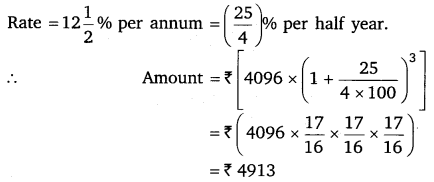Question 10.
The population of a place increased to 54,000 in 2003 at a rate of 5% per annum
(i)
find the population in 2001.
(ii) what would be its population in 2005?

Solution: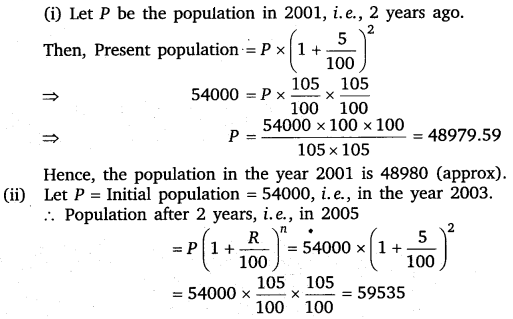Question 11.
In a Laboratory, the count of bacteria in a certain experiment was increasing at the rate of 2.5% per hour. Find the bacteria at the end of 2 hours if the count was initially 5,06,000.

Solution:
We have, P = Original count of bacteria = 506000;
Rate of increase = R = 2.5% per hour, Time = 2 hours.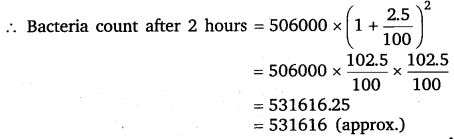Question 12.
A scooter was bought at ? 42,000. Its value depreciated at the rate of 8% per annum. Find its value after one year.

Solution:
We have, V0 = Initial value = ₹ 42000
R = Rate of depreciation = 8% p.a.